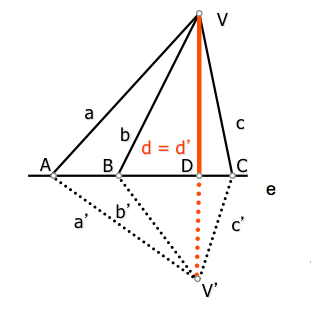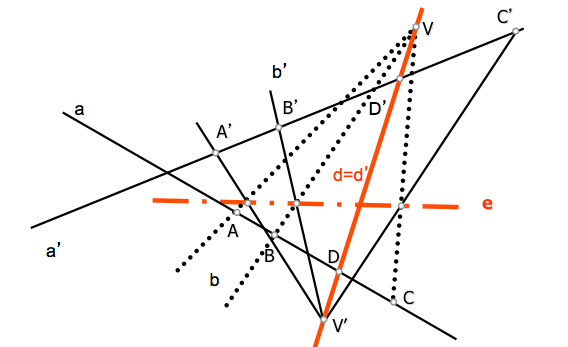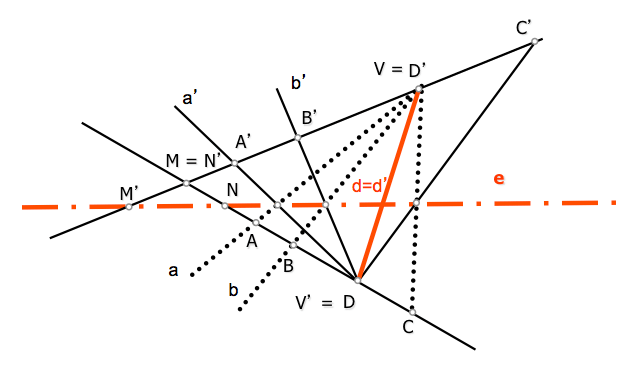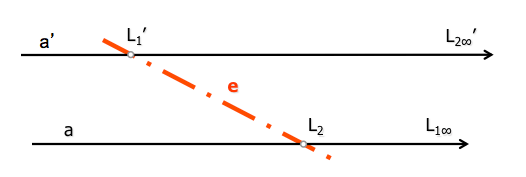# Geometría proyectiva: Projective projective axis of two seriesAbstract concepts are studied in models of projective geometry must then be translated into a set of operations for manipulating this type of transformation. The operation in prospects relationships is reduced to the concepts of belonging, so we will use these techniques to suit projective models simplify obtaining homologous elements.

The “world” points is more affordable than didactically straight dual, therefore begin our analysis with the concepts associated with rectilinear series for, later, carry out development in dual forms, straight beams.

We can consider in this study a series of basic questions that will help guide the development:

• How can we define two projective series?
• How many homologous elements are necessary to determine a projectivity
• How can we obtain homologous elements from given?

Two projective series are determined by defining three pairs of homologous points (A-A’, B-B’, C-C’), located on their respective bases.

A fourth element X of the plurality of base “to” shall have one new point X’ homologous series (projective) base “a'” so that the cross ratio of quaternions is retained determining:

(ABCX) = (A’B’C’X’)To determine the X homologue will operate using intermediate linking perspectividades Beam (projected) elements of both series.By studying the perspectivity perspectival saw two beams (prospective series with common axis section), have a dual beam is the one containing the bases (vertices) beams.

In the figure the double beam is d = d’ containing the vertices V and V’ of the beams with perspectival perspectival axis and the line.

This property is essential for finding perspectival do projective linking the two series which aim to simplify treatment, as discussed below.

Given the series of projective bases to and to’, proceed to project them for two points V and V’ determining beams with such series are perspectival. Among the countless pairs of vertices that we can use to project this series, choose two that are located in any point of a line containing two homologous series elements. The line d = d’ contains the pair D-D’ this series.

These beams straight vertices V and V 'are perspectival each other to be straight double d = d 'The line e is the beam axis perspective Vertex V.. and In ' projecting the points of the series. By varying any of the vertices of the beams (V o V’) on the d-line of, these beams will continue to be perspectival (to have a double line) but change the perspective axis position. Although the shaft change, construction for determination of homologous elements remain equally valid.

### Projective axis

By using two homologous points of the bundles as bases V and V ', these are perspectival to have a dual element. Are in the former case since we found vertices located on a line containing two homologous elements, but in this case the axis of the beams perspective is unique and depends on the pair of points selected for generating the beams perspectival. If we project therefore from A-A’ o B-B’ … the perspective axis is the same and we will call “projective axis seriesThe line and es el perspective beam axis de bases V y V’, being in turn the projective axis series de bases to and a’

The points M = N’ of intersection of the two bases have the peer axis intersection with the corresponding bases. In the case of parallel bases will become in turn points to the limits of the series.We will see later how to use the projective axis to determine pairs of homologous series elements.